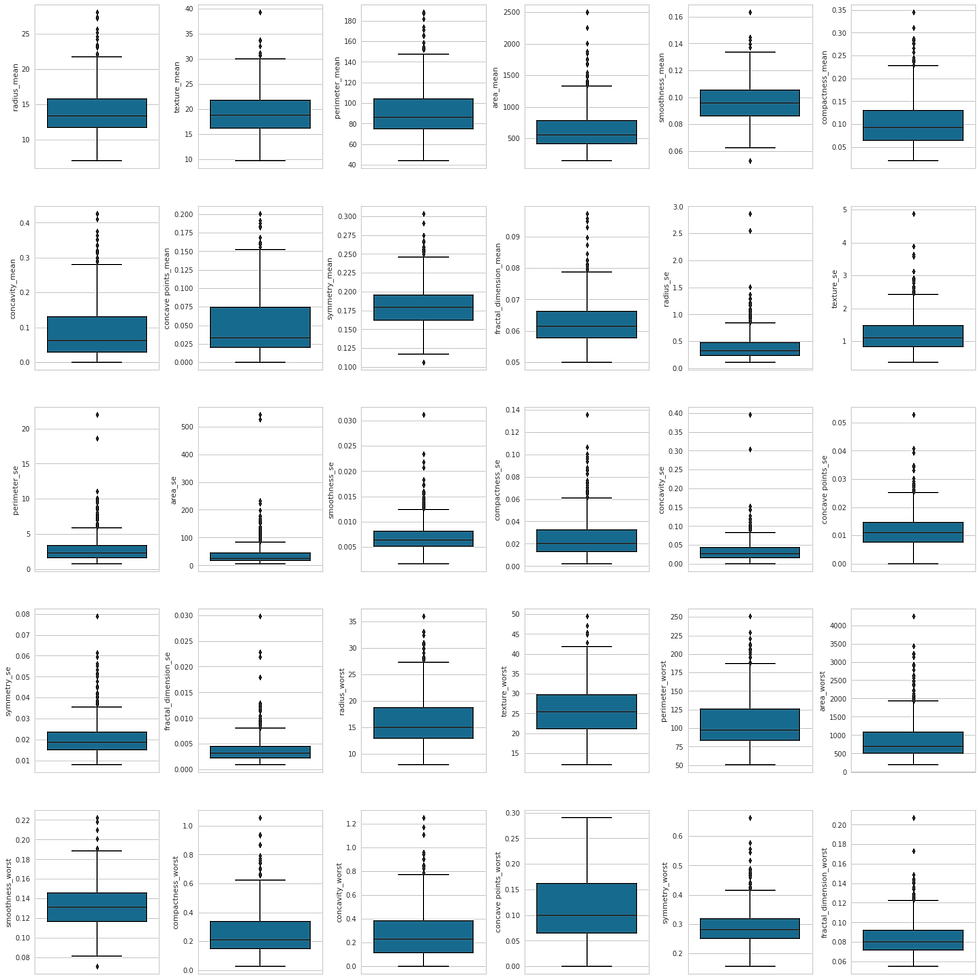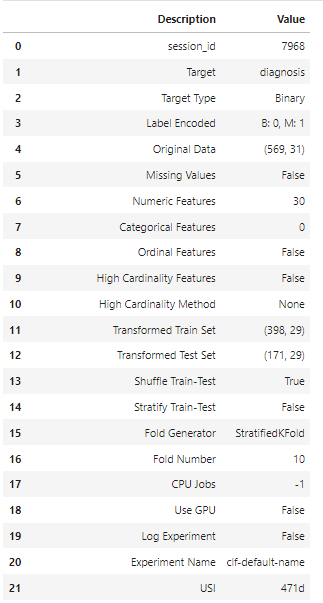top of page
• Hackers Realm

# Breast Cancer Detection Analysis using Python | Pycaret | Machine Learning Project Tutorial

Updated: Jun 5

Unlock the power of breast cancer detection analysis using Python and Pycaret! This comprehensive blog tutorial explores machine learning techniques to accurately diagnose breast cancer. Learn data preprocessing, feature engineering, and model evaluation. Dive into the world of Pycaret, a powerful machine learning library, to build and fine-tune classification models. Enhance your Python programming and machine learning skills through this step-by-step project tutorial. Join us on this transformative journey of breast cancer detection! #BreastCancerDetection #Python #Pycaret #MachineLearning #DataAnalysisBreast Cancer Detection

In this project tutorial, we will learn Breast Cancer Detection Analysis with the help of the pycaret module. It is a classification problem in machine learning. We will also explore some different methods apart from the usual workflow.

You can watch the video-based tutorial with step by step explanation down below

## Dataset Information

Features are computed from a digitized image of a fine needle aspirate (FNA) of a breast mass. They describe the characteristics of the cell nuclei present in the image.

### Attribute Information:

1. ID number

2. Diagnosis (M = malignant, B = benign)

Ten real-valued features are computed for each cell nucleus:

a) radius (mean of distances from the center to points on the perimeter) b) texture (standard deviation of grey-scale values) c) perimeter d) area e) smoothness (local variation in radius lengths) f) compactness (perimeter^2 / area - 1.0) g) concavity (severity of concave portions of the contour) h) concave points (number of concave portions of the contour) i) symmetry j) fractal dimension ("coastline approximation" - 1).

The mean, standard error and "worst" or largest (mean of the three largest values) of these features were computed for each image, resulting in 30 features. For instance, field 3 is Mean Radius, field 13 is Radius SE, field 23 is Worst Radius.

All feature values are recoded with four significant digits.

Missing attribute values: none

Class distribution: 357 benign, 212 malignant

## Install Pycaret Module

`!pip install pycaret`
• It will install all the necessary libraries for this project.

## Import modules

```import pandas as pd
import numpy as np
import seaborn as sns
import matplotlib.pyplot as plt
import warnings
from pycaret.classification import *
%matplotlib inline
warnings.filterwarnings('ignore')```
• pandas - used to perform data manipulation and analysis

• numpy - used to perform a wide variety of mathematical operations on arrays

• matplotlib - used for data visualization and graphical plotting

• seaborn - built on top of matplotlib with similar functionalities

• pycaret - import all functions for automl workflow

• %matplotlib - to enable the inline plotting.

• warnings - to manipulate warnings details filterwarnings('ignore') is to ignore the warnings thrown by the modules (gives clean results)

We will use Kaggle to load the data set.

```df = pd.read_csv('/kaggle/input/breast-cancer-wisconsin-data/data.csv')Breast Cancer Dataset
• We can drop the Id and the last column 'Unnamed:32' as it is not essential for this project.

• Diagnosis is the output column.

• The remaining are the 30 input features.

To delete unnecessary columns

```# delete unnecessary columns
df = df.drop(columns=['id', 'Unnamed: 32'], axis=1)```

Let us explore the basic information about the dataset

```# statistical info
df.describe()```Statistical Information of Dataset
• There are no missing values in this dataset.

• Later we will explore all the features with the help of Exploratory Data Analysis.

To Display the information about Dataset

```# datatype info
df.info()```Datatype Information
• Diagnosis is the output column.

• The remaining 30 features are the Input column and are NOT-NULL.

• The data type of all the attributes is float64 except Diagnosis. Thus, we can skip the preprocessing of the dataset.

## Exploratory Data Analysis

Let us explore the only output column 'Diagnosis'.

`sns.countplot(df['diagnosis'])`Distribution of Diagnosis
• The class distribution is not highly unbalanced. Therefore, we can avoid balancing the classes.

Before exploring the numerical columns, let's drop 'diagnosis' from the data frame.

`df_temp = df.drop(columns=['diagnosis'], axis=1)`

To explore the distribution of 30 numerical column, we can use subplots.

```# create dist plot
fig, ax = plt.subplots(ncols=6, nrows=5, figsize=(20, 20))
index = 0
ax = ax.flatten()

for col in df_temp.columns:
sns.distplot(df[col], ax=ax[index])
index+=1Distribution Plot of all Numerical Attributes
• Most of the attributes show Normal Distribution.

• A few of them needs to be Normalized. But as we are using 'pycaret' it will automatically take care of it.

Let us now explore the box plot of these columns.

```# create box plot
fig, ax = plt.subplots(ncols=6, nrows=5, figsize=(20, 20))
index = 0
ax = ax.flatten()

for col in df_temp.columns:
sns.boxplot(y=col, data=df, ax=ax[index])
index+=1Box Plot of all Numerical Attributes
• While dealing with large numbers of samples, we need to remove the outliers. It will improve our Model performance.

## Create and Train the Model

• We are using the pycaret module to automate the selection composition and parameterization of the models.

• If we want to add conditions and enrich the data, then we have to do it before setting up the pycaret module.

Let us set up the data for the workflow.

```# setup the data
clf = setup(df, target='diagnosis')```Pycaret Setup

You can explore more about these attributes by clicking here

• The table shows all 59 attributes.

• There are no missing values.

• While enabling GPU you can set the GPU 'True'. It will decrease the training time.

To compare the performance of the different classification models.

```# train and test the models
compare_models()```
• Since we have 500 samples the result will take some time to display.

• While dealing with more than 10,000 samples, you could also enable GPU and parallel processing to quickly layout the result.• With the help of pycaret, we can compare the available models in a tabular format.

• The table shows the result of the comparison between different models.

• It includes all the necessary matrices like Accuracy, Precision, F1-Score and Kappa etc.

The results indicates that CatBoost is the best Model.

Let us train the model with the CatBoost Classifier.

```# select the best model
model = create_model('catboost')```• The CatBoost shows an overall good result.

Let us now fine-tune our model.

```# hyperparameter tuning
best_model = tune_model(model)```• The mean result is decreased compared to the default parameters.

• However the precision has improved, we cannot finalize with these hyper tuned models.

## Evaluate The Model

`evaluate_model(best_model)`• Running this code will allow us to choose the graph of all parameters.

Let us plot the result using Confusion Matrix

```# plot the results
plot_model(estimator=best_model, plot='confusion_matrix')```• The confusion matrix displays the actual and predicted classes.

• We observe 2 and 4 in the left diagonal representing the fewer errors in the confusion matrix.

## Final Thoughts

• We can finalize our project by considering both the AUC and the precision.

• The Precision is around 96%, and the AUC outcome is almost 99.5%, which is a good result.

• In this project, the processes are carried out by the pycaret module, and the code required is less.

• As a beginner, you can explore data efficiently using pycaret.

In this project tutorial, we have explored all the basic operations in the pycaret module. You can also explore NLP and Regression using the same technique.

Get the project notebook from here